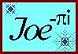Helix Calculator
 Radius = Height = Angle in Plan =
 Helix Data
 Cylinder Circumference = Helix Length = Unit Rise = Pitch Angle: Degrees = Handrail Radius =
 Data for Specified Section
 Fraction of Total = Length of Circle Arc = Helix Section Length =